Courses

# RD Sharma Solutions Ex-14.4, (Part - 1), Quadrilaterals, Class 9, Maths Class 9 Notes | EduRev

## Class 9 : RD Sharma Solutions Ex-14.4, (Part - 1), Quadrilaterals, Class 9, Maths Class 9 Notes | EduRev

The document RD Sharma Solutions Ex-14.4, (Part - 1), Quadrilaterals, Class 9, Maths Class 9 Notes | EduRev is a part of the Class 9 Course RD Sharma Solutions for Class 9 Mathematics.
All you need of Class 9 at this link: Class 9

Q.1. In ΔABC, D, E and F are, respectively the mid points of BC, CA and AB. If the lengths of sides AB, BC and CA are 7cm, 8cm and 9cm, respectively, find the perimeter of ΔDEF.

Solution: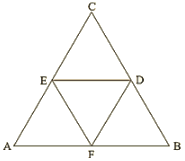Given that,

AB = 7cm, BC = 8cm, AC = 9cm

In ∆ABC,

F and E are the mid points of AB and AC.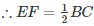Similarly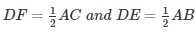Perimeter of ∆DEF = DE + EF + DF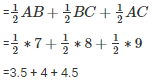=12cm

∴ Perimeterof ΔDEF = 12cm

Q.2. In a ΔABC, ∠A=500, ∠B=600 and ∠C=700. Find the measures of the angles of the triangle formed by joining the mid-points of the sides of this triangle.

Solution: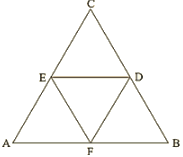In ΔABC,

D and E are mid points of AB and BC.

By Mid point theorem,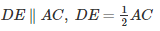F is the midpoint of AC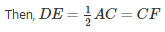DE∥AC,DE = CF

Hence DECF is a parallelogram.

∴∠C =∠D = 700        [Opposite sides of a parallelogram]

Similarly

BEFD is a parallelogram, ∠B =∠F = 600

ADEF is a parallelogram, ∠A = ∠E = 500

∴ Angles of ΔDEF are

∠D = 700,∠E = 500,∠F = 600

Q.3. In a triangle, P, Q and R are the mid points of sides BC, CA and AB respectively. If AC = 21cm, BC = 29cm and AB = 30cm, find the perimeter of the quadrilateral ARPQ.

Solution: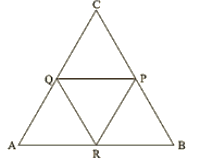In ΔABC,

R and P are mid points of AB and BC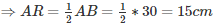[By Mid point Theorem]

[A pair of side is parallel and equal]

RP∥AQ,RP = AQ

Therefore, RPQA is a parallelogram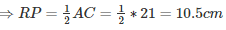AR = QP = 15cm      [Opposite sides are equal]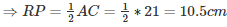RP = AQ = 10.5cm           [Opposite sides are equal ]

Now,

Perimeter of ARPQ = AR + QP + RP +AQ

= 15 +15 +10.5 +10.5

= 51cm

Q.4. In a ΔABC median AD is produced to X such that AD = DX. Prove that ABXC is a parallelogram.

Solution: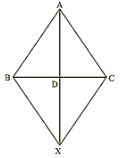In a quadrilateral ABXC, we have

BD = DC [Given]

So, diagonals AX and BC bisect each other.

Therefore, ABXC is a parallelogram.

Q.5. In a ΔABC, E and F are the mid-points of AC and AB respectively. The altitude AP to BC intersects FE at Q. Prove that AQ = QP.

Solution: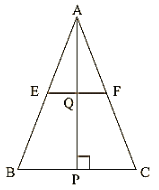In a ΔABC

E and F are mid points of AB and AC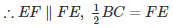[By mid point theorem]

In  ΔABP

F is the mid-point of AB and ∴ FQ∥BP[∵EF∥BP]

Therefore, Q is the mid-point of AP               [By mid-point theorem]

Hence, AQ = QP.

Q6. In a ΔABC, BM and CN are perpendiculars from B and C respectively on any line passing through A. If L is the mid-point of BC, prove that ML = NL.

Solution: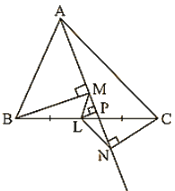Given that,

In ΔBLMandΔCLN

∠BML=∠CNL= 900

BL = CL    [L is the mid-point of BC]

∠MLB = ∠NLC     [Vertically opposite angle]

∴ΔBLM = ΔCLN

∴LM = LN           [corresponding parts of congruent triangles]

Q.7. In figure 14.95, Triangle ABC is a right angled triangle at B. Given that AB = 9cm, AC = 15cm and D, E are the mid-points of the sides AB and AC respectively, calculate

(i) The length of BC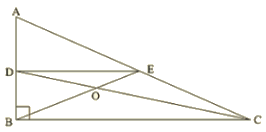Solution:

In ΔABC,∠B=  900,

By using Pythagoras theorem

AC2 = AB2 + BC2

⇒152 = 92 + BC2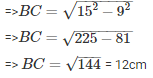D and E are mid-points of AB and AC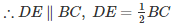[By mid−point theorem]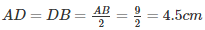[∵D is the mid−point of AB]]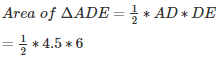=13.5cm2

Q.8. In figure 14.96, M, N and P are mid-points of AB, AC and BC respectively. If MN = 3cm, NP = 3.5cm and MP = 2.5cm, calculate BC, AB and AC.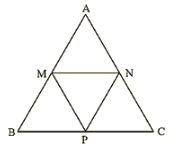Solution:

Given MN = 3cm, NP = 3.5cm and MP = 2.5cm.

To find BC, AB and AC

In ΔABC

M and N are mid-points of AB and AC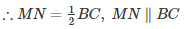[By mid−point theorem]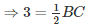⇒ 3∗2 = BC

⇒ BC = 6cm

Similarly

AC = 2MP = 2 (2.5) = 5cm

AB = 2 NP = 2 (3.5) = 7cm

Q.9. ABC is a triangle and through A, B, C lines are drawn parallel to BC, CA and AB respectively intersecting at P, Q and R. Prove that the perimeter of ΔPQR is double the perimeter of ΔABC.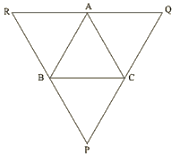Solution:

Clearly ABCQ and ARBC are parallelograms.

Therefore, BC = AQ and BC = AR

⇒AQ = AR

⇒A is the mid-point of QR

Similarly B and C are the mid points of PR and PQ respectively.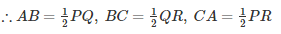⇒ PQ = 2AB, QR = 2BC and PR = 2CA

⇒ PQ + QR + RP = 2 (AB + BC + CA)

⇒ Perimeter of ΔPQR = 2 (perimeter of ΔABC)

Q.10. In figure 14.97, BE⊥AC, AD is any line from A to BC intersecting BE in H. P, Q and R are respectively the mid-points of AH, AB and BC. Prove that ∠PQR=900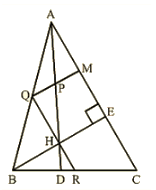Solution:

Given,

BE⊥AC and P, Q and R are respectively mid-point of AH, AB and BC.

To prove: ∠PQR = 900

Proof: In ΔABC, Q and R are mid-points of AB and BC respectively.

∴ QR∥AC…..(i)

In ΔABH, Q and P are the mid-points of AB and AH respectively

∴ QP∥BH….(ii)

But, BE⊥AC

Therefore, from equation (i) and equation (ii) we have,

QP⊥QR

⇒∠PQR = 900

Hence Proved

Offer running on EduRev: Apply code STAYHOME200 to get INR 200 off on our premium plan EduRev Infinity!

91 docs

,

,

,

,

,

,

,

,

,

,

,

,

,

,

,

,

,

,

,

,

,

,

,

,

,

,

,

,

,

,

,

,

,

;